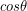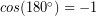# 10.4: Doing Work

$$\newcommand{\vecs}{\overset { \rightharpoonup} {\mathbf{#1}} }$$ $$\newcommand{\vecd}{\overset{-\!-\!\rightharpoonup}{\vphantom{a}\smash {#1}}}$$$$\newcommand{\id}{\mathrm{id}}$$ $$\newcommand{\Span}{\mathrm{span}}$$ $$\newcommand{\kernel}{\mathrm{null}\,}$$ $$\newcommand{\range}{\mathrm{range}\,}$$ $$\newcommand{\RealPart}{\mathrm{Re}}$$ $$\newcommand{\ImaginaryPart}{\mathrm{Im}}$$ $$\newcommand{\Argument}{\mathrm{Arg}}$$ $$\newcommand{\norm}{\| #1 \|}$$ $$\newcommand{\inner}{\langle #1, #2 \rangle}$$ $$\newcommand{\Span}{\mathrm{span}}$$ $$\newcommand{\id}{\mathrm{id}}$$ $$\newcommand{\Span}{\mathrm{span}}$$ $$\newcommand{\kernel}{\mathrm{null}\,}$$ $$\newcommand{\range}{\mathrm{range}\,}$$ $$\newcommand{\RealPart}{\mathrm{Re}}$$ $$\newcommand{\ImaginaryPart}{\mathrm{Im}}$$ $$\newcommand{\Argument}{\mathrm{Arg}}$$ $$\newcommand{\norm}{\| #1 \|}$$ $$\newcommand{\inner}{\langle #1, #2 \rangle}$$ $$\newcommand{\Span}{\mathrm{span}}$$$$\newcommand{\AA}{\unicode[.8,0]{x212B}}$$

## Work

So far we have thought about decreasing force during collisions by increasing the time over which the force is applied. Instead of using the impulse, which combines average force and time over which it is applied:

(1)We can instead combine average force with the distance over which it is applied to define a quantity known as the work.

(2)Thetells us whether the work is transferring energy into or out of a particular object:

1. A force applied to an object in the opposite direction to its motion will tend to slow it down, and thus would transfer kinetic energy out of the object. With energy leaving the object, the work done on the object should be negative. The angle between the object’s motion and the force in such a case is 180° and, so that checks out.
2. A force applied to an object in the same direction to its motion will tend to cause it to speed up, and thus would transfer kinetic energy in to the object. With energy entering the object, the work done on the object should be positive. The angle between the object’s motion and the force in such a case is 0° andso that also checks out.
3. Finally, if a force acts perpendicular to an objects motion it can only change its direction of motion, but won’t cause it to speed up or slow down, so the kinetic energy doesn’t change. That type of force should do zero work. The angle between the object’s motion and the force in such a case is 90° andso once again, thein the work equation gives the required result. For more on this particular type of situation read the chapter on weightlessness at the end of this unit.Insuknawr, or Rod Pushing Sport is an indigenous game of Mizoram, one of the North Eastern States of India. A force applied in the same direction as an objects motion does positive work. A force applied in the opposite direction to motion does negative work. Image adapted from <a href=”commons.wikimedia.org/wiki/File:Insuknawr(Rod_Pushing_Sport).JPG”>Insuknawr (Rod Pushing Sport by H. Thangchungnunga via Wikimedia Commons

We see that work and kinetic energy both have units of Nm (or J). Work is a quantity of energy, however is not a type of energy. Rather, work is an amount of energy transferred by the application of a force over a distance. Doing work is the act of transferring energy from one form to another and/or one object to another. The sign of the work indicates if energy is coming in or going out, rather than indicating a direction in space like the signs on a vector such as force and velocity. Therefore, we have not made work (W) bold in the previous equation because it is not a vector. Also, when calculating work theaccounts for the force direction so we only use the size of the force (F) in the equation, which is why we have not made force bold either.

#### Everyday Example

Let’s apply this concept to our crumple zone example. The crumple zone increases the distance over which the force on the car, from the wall, is applied. That force on the car points in the opposite direction of its motion, thus it will be a negative work that transfers energy out of the car (no work is “absorbed” here, just transferred from kinetic energy to another type). In order to stop, the KE of the car must change from its initial value to zero, therefore the amount of work that needs to be done is equal to the total amount of KE the car had to begin with. The initial KE is determined by the speed and mass of the car, but that work can be done by a larger force over a shorter distance or a smaller force over a longer distance. The crumple zone ensures the force is applied over a longer distance thus smaller force. (If the car didn’t crumple at all, but only received a small dent, then would only be a few centimeters).

The work equation gives the correct work done by a force, no matter the angle between the direction of force and the direction of motion, even if the force points off at some angle other than 0°, 90°, or 180°. In such a case, some part of the force will be doing work and some part won’t, but thetells us just how much of the force vector is contributing to work.

#### Reinforcement Exercises

How much work is done by the larger child pulling the smaller one for a distance of 30.0 m in a wagon as shown?A child pulls another in a wagon, exerting a force at an angle relative to the direction of motion. “This work” by BC Open Textbooks

1. Adapted from Insuknawr (Rod Pushing Sport by H. Thangchungnunga [CC BY-SA 3.0 (https://creativecommons.org/licenses/by-sa/3.0) or GFDL (http://www.gnu.org/copyleft/fdl.html)], from Wikimedia Commons